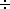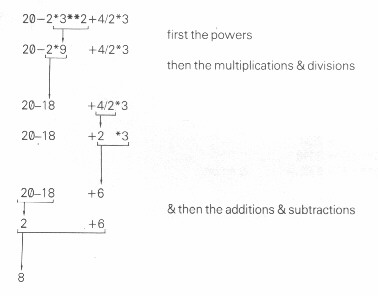Chapter 4 - The Sinclair ZX81 as a calculator

Turn the computer on. You can now use it as a calculator, along the lines of chapter 2: type PRINT, then whatever it is that you want working out, & then NEWLINE. (We shan't usually bother to tell you to type NEWLINE.)

As you would hope, the ZX81 can not only add, but also subtract, multiply using a star * instead of the usual times sign - this is fairly common on computers) & divide (using / instead of). Try these out.

+, -, * and / are operations, & the numbers they operate on are their operands.

The computer can also raise one number to the power of another using the operation ** (Shifted H. Do not type * - shifted B - twice): type

PRINT 2**3 (Remember the NEWLINE.)

& you will get the answer 8 (2 raised to the power 3, or 23, or 2 cubed)

The ZX81 will also work out combinations of the operations. For instance.

PRINT 20-2*3**2+4/2*3

gives the answer 8. It goes all round the houses to get this, because first it works out all the powers (**) in order from left to right, & then all the multiplications & divisions (* & /), again from left to right, & then the additions & subtractions (+ & -), yet again from left to right. Thus our example is worked out in the following stages:We formalize this by giving each operation a priority, a number between 1 & 16. The operations with highest priority are evaluated first, & operations with equal priority are evaluated in order from left to right.

 ** has priority 10 * and / have priority 8 + & - have priority 6

When - is used to negate something, as when you write -1, then it has priority 9. (This is unary minus, as opposed to the binary minus in 3-1: a unary operation has one operand, while a binary operation has two. Note that on the ZX81 you cannot use + as a unary operation.)

This order is absolutely rigid, but you can circumvent it by using brackets: anything in brackets is evaluated first & then treated as a single number, so that

PRINT 3*2+2

gives the answer 6+2 = 6, but

PRINT 3*(2+2)

gives the answer 3*4 = 12.

A combination like this is called an expression - in this case, an arithmetic or numeric expression because the answer is a number. In general, whenever the computer is expecting a number from you, you can give it an expression instead and it will work out the answer.

You can write numbers with decimal points (use the full stop), & you can also use scientific notation - as is quite common on pocket calculators. In this, after an ordinary number (with or without a decimal point), you can write an exponent part consisting of the letter E, then maybe + or -, & then a number without a decimal point. The E here means '*10**' ('times ten to the power of'), so that

 2.34 = 2.34 * 10**0  = 2.34 2340 = 2.34 * 10**3  = 2340 0.0234 = 2.34 * 10**-2 = 0.0234 & so on.

(Try printing these out on the ZX81.)

The easiest way of thinking of this is to imagine the exponent part shifting the decimal point along to the right (for a positive exponent) or to the left (for a negative exponent).

You can also print more than one thing at once, separating them either with commas (,) or semicolons (; or shifted X). If you use a comma, then the next number will be displayed starting either at the left hand margin, or in the middle of the line in the 16th column. If you use a semicolon, then the next number will be displayed immediately following the last one.

Try

PRINT 1;2;3;4;5;6;7;8;9;10

&

PRINT 1,2,3,4,5,6,7,8,9,10

to see the differences. You can mix commas & semicolons within a single PRINT statement if you want.

Summary

Statements: PRINT, with commas & semicolons

Operations: +,-,*,/,**

Expressions, scientific notation

Exercises

1. Try

PRINT 2.34E0

PRINT 2.34E1

PRINT 2.34E2

and so on up to

PRINT 2.34E15

You will see that after a while the ZX81 also starts using scientific notation. This is because it never takes more than 14 spaces to write a number in. Similarly, try

PRINT 2.34E-1

PRINT 2.34E-2

& so on.

2. Try

PRINT 1,,2,,3,,,4,,,,5

A comma always moves you on a bit for the next number. Now try

PRINT 1;;2;;3;;;4;;;;5

Why is a string of semicolons no different from a single one?

3. PRINT gives only 8 significant digits. Try

PRINT 4294967295, 4294967295 -429E7

This proves that the computer can hold all the digits of 4294967295, even though it is not prepared to display them all at once.

4. If you've got some log tables, then test out this rule:

Raising 10 to the power of a number is the same as taking the antilog of that number.

For instance, type

PRINT 10**0.3010

& look up the antilog of 0.3010. Why are the answers not exactly equal?

5. The ZX81 uses floating point arithmetic, which means that it keeps separate the digits of a number (its mantissa) and the position of the point (the exponent). This is not always exact, even for whole numbers. Type

PRINT 1E10+1-1E10,1E10-1E10+1

Numbers are held to about 9 1/2 digits accuracy, so 1E10 is too big to be held exactly right. The inaccuracy (actually about 2) is more than 1, so the numbers 1E10 & 1E10+1 appear to the computer to be equal.

For an even more peculiar example, type

PRINT 5E9+1-5E9

Here the inaccuracy in 5E9 is only about 1, & the 1 to be added on in fact gets rounded up to 2. Here the numbers 5E9+1 & 5E9+2 appear to the computer to be equal.

The larger integer (whole number) that can be held completely accurately is 232-1 (4,294,967,295).

Previous: Chapter 3    Next: Chapter 5